NEET  >  Test: Stoichiometry & Stoichiometric Calculations (NCERT)

# Test: Stoichiometry & Stoichiometric Calculations (NCERT) - NEET

Test Description

## 25 Questions MCQ Test Chemistry Class 11 - Test: Stoichiometry & Stoichiometric Calculations (NCERT)

Test: Stoichiometry & Stoichiometric Calculations (NCERT) for NEET 2023 is part of Chemistry Class 11 preparation. The Test: Stoichiometry & Stoichiometric Calculations (NCERT) questions and answers have been prepared according to the NEET exam syllabus.The Test: Stoichiometry & Stoichiometric Calculations (NCERT) MCQs are made for NEET 2023 Exam. Find important definitions, questions, notes, meanings, examples, exercises, MCQs and online tests for Test: Stoichiometry & Stoichiometric Calculations (NCERT) below.
Solutions of Test: Stoichiometry & Stoichiometric Calculations (NCERT) questions in English are available as part of our Chemistry Class 11 for NEET & Test: Stoichiometry & Stoichiometric Calculations (NCERT) solutions in Hindi for Chemistry Class 11 course. Download more important topics, notes, lectures and mock test series for NEET Exam by signing up for free. Attempt Test: Stoichiometry & Stoichiometric Calculations (NCERT) | 25 questions in 25 minutes | Mock test for NEET preparation | Free important questions MCQ to study Chemistry Class 11 for NEET Exam | Download free PDF with solutions
 1 Crore+ students have signed up on EduRev. Have you?
Test: Stoichiometry & Stoichiometric Calculations (NCERT) - Question 1

### H2O2 acts as both oxidising as well as reducing agent its product is H2O, when act as oxidising agent and its product is 02 when act as of reducing agent. The strength of '10P means one liter of H2O2 solution on decomposition at S.T.P. condition liberate 10 liters of oxygen gas (H2O → H2O + 1/2 O2) 15 gm Mn04)2 sample containing inert impurity is completely reacting with 100 ml of '11.2 V' H2O2, then what will be the % purity of Ba (Mn04)2 in the sample?            (Atomic mass Ba = 137, Mn = 55)

Detailed Solution for Test: Stoichiometry & Stoichiometric Calculations (NCERT) - Question 1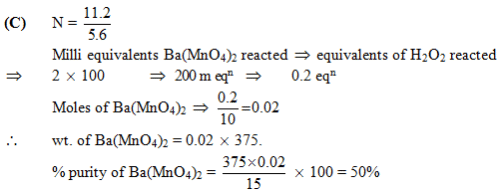Test: Stoichiometry & Stoichiometric Calculations (NCERT) - Question 2

### 1.2 gm of carbon is burnt completely in oxygen (limited supply) to produce CO and CO2. This mixture of gases is treated with solid I2O5 (to know the amount of CO produced), the liberated iodine required 120 ml of 0.1 M hypo solution for complete titration. The % of carbon converted into CO is:

Detailed Solution for Test: Stoichiometry & Stoichiometric Calculations (NCERT) - Question 2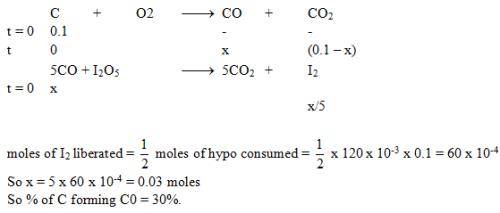Hence, the correct answer is Option D.

Test: Stoichiometry & Stoichiometric Calculations (NCERT) - Question 3

### Nitrogen (N), phosphorus (P) and Potassium (K) are the main nutrients in plant fertilizers. According to an industry convention, the numbers on the label refer to the mass % of N, P2O5 and K2O in that order calculate the N : P : K ratio of a 30 : 10 : 10, fertilizer in terms of moles of each element and express it as x: y : 1.

Detailed Solution for Test: Stoichiometry & Stoichiometric Calculations (NCERT) - Question 3

Molar mass of N is 14.0067 g/mol.
Molar mass of P2O5 is 30.973762*205 = 6349.62121 g/mol.
Molar mass of K2O is 39.0983*20 = 781.966 g/mol
Ratio of masses is 30:10:10
Therefore for each 50 g of fertilizer:
For N: 30 g / 14.0067 g/mol = 30/14.0067 moles
For P2O5: 10 g / 6349.62121 g/mol = 10/6349.62121 moles
For K2O: 10 g / 781.966 g/mol = 10/781.966 moles
Ratio of elements in terms of moles:
30/14.0067 : 10/6349.62121 : 10/781.966
30/14.0067*781.966/10 : 10/6349.62121*781.966/10 : 10/781.966*781.966/10
167.484 : 0.123 : 1.0

Test: Stoichiometry & Stoichiometric Calculations (NCERT) - Question 4

In what ratio should a 15% solution of acetic acid be mixed with a 3% solution of the acid to prepare a 10% solution(all percentages are mass/mass percentages):

Detailed Solution for Test: Stoichiometry & Stoichiometric Calculations (NCERT) - Question 4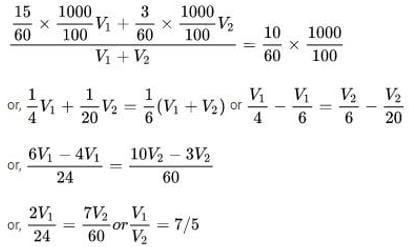Test: Stoichiometry & Stoichiometric Calculations (NCERT) - Question 5

105 ml of pure water at 4°C saturated with NH3 gas yielded a solution of density 0.9 g/ml and containing 30% NH by mass. Find the volume of resulting NH3 solution.

Detailed Solution for Test: Stoichiometry & Stoichiometric Calculations (NCERT) - Question 5

Given -
volume of water = 105 mL
density of solution = 0.9 g/mL
Calculation -
Since ammonia dissolved in water is 30% by weight, then using mass % formula, we can calculate the amount of ammonia in water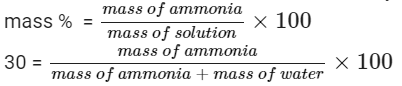At 4 degree C, density of water  = 0.999 g/mL
since density = mass /volume
0.999  = mass/ 105
mass of water = 104.895 g
​now, let mass of ammonia be x.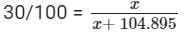30x + 3146.85 = 100x
70x = 3146.85
x = 44.955 g
Thus, ammonia dissolved in 105 mL water is 44.955 g
Therefore, total mass of solution = mass of ammonia + mass of water
Mass of solution = 44.955 + 104.895
Mass of solution = 149.85 g
So,density of solution = mass of solution / volume of solution
​0.9 g/mL  = 149.85 g / Volume of solution
Volume of solution = 149.85/ 0.9
Volume of solution = 166.5 mL

Test: Stoichiometry & Stoichiometric Calculations (NCERT) - Question 6

The equivalent mass of H3BO3 (M = Molar mass of H3BO3) in its reaction with NaOH to from Na2B4O7 is equal to –

Detailed Solution for Test: Stoichiometry & Stoichiometric Calculations (NCERT) - Question 6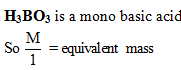Test: Stoichiometry & Stoichiometric Calculations (NCERT) - Question 7

X gram of pure As2S3 is completely oxidised to respective highest oxidation states by 50 ml of 0.1 M hot acidified KMnO4 then x mass of As2S3 taken is : (Molar mass of As2S3 = 246)

Detailed Solution for Test: Stoichiometry & Stoichiometric Calculations (NCERT) - Question 7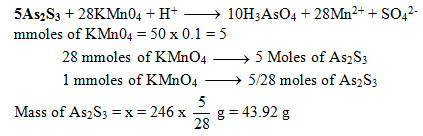Test: Stoichiometry & Stoichiometric Calculations (NCERT) - Question 8

The number of moles of ferrous oxalate oxidised by one mole of KMnO4 is

Detailed Solution for Test: Stoichiometry & Stoichiometric Calculations (NCERT) - Question 8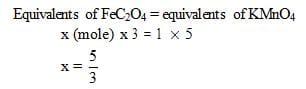Test: Stoichiometry & Stoichiometric Calculations (NCERT) - Question 9

How many moles of KMnO4 are needed a mixture of 1 mole of each FeSO4 & FeC2O4 in acidic medium

Detailed Solution for Test: Stoichiometry & Stoichiometric Calculations (NCERT) - Question 9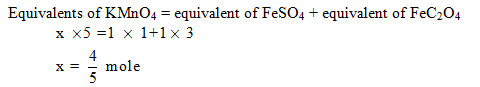Test: Stoichiometry & Stoichiometric Calculations (NCERT) - Question 10

In the reaction

Na2S2O3 + 4C12 + 5H2O → Na2SO4 + H2S04 + 8HCI

the equivalent weight of Na2 S2 O3 will be

(M= molecular weight of Na2S2O3)

Detailed Solution for Test: Stoichiometry & Stoichiometric Calculations (NCERT) - Question 10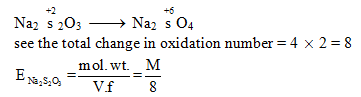Test: Stoichiometry & Stoichiometric Calculations (NCERT) - Question 11

C2H4 + xO2 -> 2CO2 + yH2O, what is the value of x + y?

Detailed Solution for Test: Stoichiometry & Stoichiometric Calculations (NCERT) - Question 11

The balance combustion equation is C2H4 + 3O2 -> 2CO2 + 2H2O, => x = 3, y = 2, => x + y = 5.

Test: Stoichiometry & Stoichiometric Calculations (NCERT) - Question 12

The following equations are balanced atomwise and chargewise.

(i) Cr2O72- + 8H+ + 2H2O2 → 2Cr3+ + 7H2O + 2O2

(ii) Cr2O72- + 8H+ + 5H2O2→ 2Cr3+ + 9H2O + 4O2

(iii) Cr2O72- + 8H+ + 7H2O2→ 2Cr+ + 11H2O + 5O2

The precise equationl equations representing the oxidation of H2O2 is/are

Detailed Solution for Test: Stoichiometry & Stoichiometric Calculations (NCERT) - Question 12

The correct answer is option A
Cr2O72- converts into Cr3+ in acidic medium I.e. in H+ medium.
First balance the Cr atom on both sides and then Oxygen atom. H+ is in excess due to acidic medium.
Add H+ as +ve charge to balance the charge on both sides.

Test: Stoichiometry & Stoichiometric Calculations (NCERT) - Question 13

An excess of NaOH was added to 100 mL of a ferric chloride solution. This caused the precipitation of 1,425 g of Fe(OH)3. Calculate the normality of the ferric chloride solution

Detailed Solution for Test: Stoichiometry & Stoichiometric Calculations (NCERT) - Question 13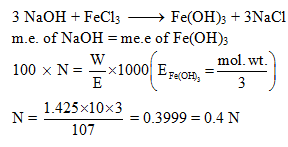Test: Stoichiometry & Stoichiometric Calculations (NCERT) - Question 14

In the reaction CrO5 + H2SO4 →Cr2(SO4)3 + H2O + O2 one mole of CrO5 will liberate how many moles of O2

Detailed Solution for Test: Stoichiometry & Stoichiometric Calculations (NCERT) - Question 14

The balanced chemical reaction is,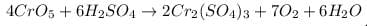From the balanced chemical reaction, we conclude that

As, 4 moles of CrO5 react to give 7 moles of O2

So, 1 mole of CrO5 react to give 7/4 moles of O2

Test: Stoichiometry & Stoichiometric Calculations (NCERT) - Question 15

0.4g of a polybasic acid HnA (all the hydrogens are acidic) requires 0.5g of NaOH for complete neutralization. The number of replaceable hydrogen atoms in the acid and the molecular weight of 'A' would be : (Molecular weight of the acid is 96 gms.)

Detailed Solution for Test: Stoichiometry & Stoichiometric Calculations (NCERT) - Question 15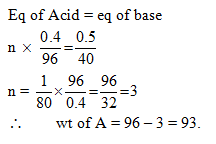Test: Stoichiometry & Stoichiometric Calculations (NCERT) - Question 16

A solution of Na2S2O3 is standardized iodimetrically against 0.1262 g of Mr% This process requires 45 mL of the Na2 S203 solution. What is the strength of the Na2S2O3?

Detailed Solution for Test: Stoichiometry & Stoichiometric Calculations (NCERT) - Question 16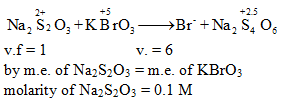Test: Stoichiometry & Stoichiometric Calculations (NCERT) - Question 17

25.0 g of FeSO4.7H2O was dissolved in water containing dilute H2SO4, and the volume was made up to 1.0 L. 25.0 mL of this solution required 20 mL of an N/10 KMnO4 solution for complete oxidation. The percentage of FeSO4. 7H2O in the acid solution is

Detailed Solution for Test: Stoichiometry & Stoichiometric Calculations (NCERT) - Question 17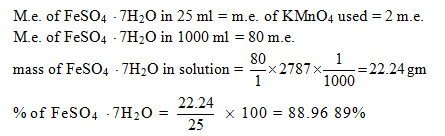Test: Stoichiometry & Stoichiometric Calculations (NCERT) - Question 18

1.0 mol of Fe reacts completely with 0.65 mol of O2 to give a mixture of only Fe0 and Fe2O3. The mole ratio of ferrous oxide to ferric oxide is

Detailed Solution for Test: Stoichiometry & Stoichiometric Calculations (NCERT) - Question 18

2Fe + O2 →→ 2FeO
​4Fe + 3O2 →→2Fe2O3
Let x moles  of Fe reacts to produce FeO
Thus amount of Fe reacted to produce Fe2O3 = 1-x  Moles
Now
2 Moles of Fe reacts to produce FeO with = 1 moles of O2
1 moles of Fe reacts ​ to produce FeO with = 1/2 moles of O2
x moles of Fe ​ to produce FeO with = 0.5x moles of O2
4 moles of Fe reacts to produce Fe2O3 with =  3 moles of O2
1-x moles of Fe reacts to produce Fe2O3 = (3/4) x (1-x) Moles of O2
EQUATION :
1)  0.5x + 3/4(1−x) =0.65 moles of O2
Thus
x = 0.4 moles
2 Moles of Fe produces = 2 mole of FeO
0.4 moles of Fe produces = 0.4 moles of FeO
4 moles of Fe produces = 2 moles of  Fe2O3
(1-0.4 )moles of Fe produces = (1/2) x 0.6 Moles = 0.3 moles of Fe2O3
Thus
FeO/Fe2O3 = 0.4/0.3 = 4/3

Test: Stoichiometry & Stoichiometric Calculations (NCERT) - Question 19

25 mL of a solution containing HCf and H2S04 required 10 mL of a 1 N NaOH solution for neutralization. 20 mL of the same acid mixture on being treated with an excess of AgNO3 gives 0.1425 g of AgCf. The normality of the HC and the normality of the H2S04 are respectively

Detailed Solution for Test: Stoichiometry & Stoichiometric Calculations (NCERT) - Question 19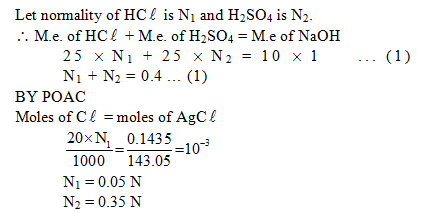Test: Stoichiometry & Stoichiometric Calculations (NCERT) - Question 20

If 10 gm of V2O5 is dissolved in acid and is reduced to V2+ by zinc metal, how many mole of I2 could be reduced by the resulting solution if it is further oxidised to VO2+ ions ?

[Assume no change in state of Zn2+ions] (V = 51, 0 = 16, I = 127) :

Detailed Solution for Test: Stoichiometry & Stoichiometric Calculations (NCERT) - Question 20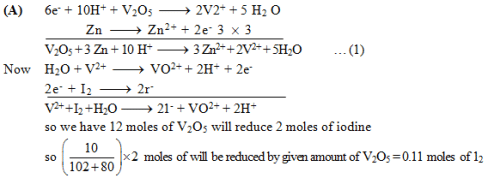Test: Stoichiometry & Stoichiometric Calculations (NCERT) - Question 21

0.70 g of mixture (NH4)2 SO4 was boiled with 100 mL of 0.2 N NaOH solution till all the NH3(g) evolved and get dissolved in solution itself. The remaining solution was diluted to 250 mL. 25 mL of this solution was neutralized using 10 mL of a 0.1 N H2SO4 solution. The percentage purity of the (NH4)2 SO sample is

Detailed Solution for Test: Stoichiometry & Stoichiometric Calculations (NCERT) - Question 21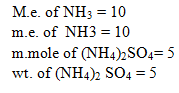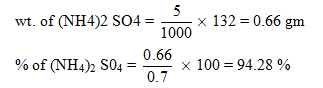Test: Stoichiometry & Stoichiometric Calculations (NCERT) - Question 22

A mixed solution of potassium hydroxide and sodium carbonate required 15 mL. of an N/20 HCI solution when titrated with phenolphthalein as an indicator. But the same amount of the solution when titrated with methyl orange as an indicator required 25 mL of the same acid. The amount of KOH present in the solution is

Detailed Solution for Test: Stoichiometry & Stoichiometric Calculations (NCERT) - Question 22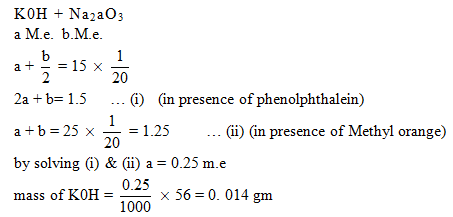Test: Stoichiometry & Stoichiometric Calculations (NCERT) - Question 23

The percentage of copper in a copper(II) salt can be determined by using a thiosulphate titration. 0.305 gm of a copper(II) salt was dissolved in water and added to, an excess of potassium iodide solution liberating iodine according to the following equation

2Cu2 (aq) + 4I (aq)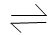2CuI(s) + I2(aq)

The iodine liberated required 24.5cm3 of a 0.100 mole dm-3 solution of sodium thiosulphate

2S2032- (aq) + I2(aq) → 2I (aq) + S4062- (aq)

the percentage of copper, by mass in the copper (ll) salt is. [Atomic mass of copper = 63.5]

Detailed Solution for Test: Stoichiometry & Stoichiometric Calculations (NCERT) - Question 23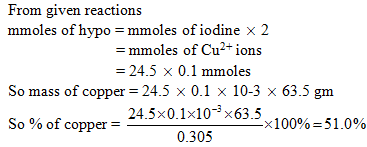Test: Stoichiometry & Stoichiometric Calculations (NCERT) - Question 24

KIO3 reacts with KI to liberate iodine and liberated Iodine is titrated with standard hypo solution, The reactions are

1. IO3 + I →I2                (valency factor = 5/3)

2. I2 + S2O32– → S4O62– + I–        (valency factor = 2)

meq of hypo = meq of I2 = meq of IO3 + meq of I

IO3 react with I ⇒ meq of IO3 = meq of I

Statement-1 : meq of hypo = 2 x meq of IO3

Statement-2 : valency factor of I2 in both the equation are different therefore we cannot equate milliequivalents in sequence

Detailed Solution for Test: Stoichiometry & Stoichiometric Calculations (NCERT) - Question 24

The correct answer is option A
Milliequivalent of IO3​ is equal to milliequivalent of I.
Milliequivalent of hypo is equal to milliequivalent of IO3​ plus milliequivalent of I.
The milliequivalent of hypo is twice the milliequivalent of IO3​. It is also twice the milliequivalent of I.

Test: Stoichiometry & Stoichiometric Calculations (NCERT) - Question 25

In the reaction:
2 C2H6 + 7 O2 → 4 CO2 + 6 H2O
how many moles of CO2 are formed when 1 mole of O2 is consumed?

Detailed Solution for Test: Stoichiometry & Stoichiometric Calculations (NCERT) - Question 25

1 mole O2 x (4 mole CO2 / 7 mole O2 ) = 4/7 mole CO2

## Chemistry Class 11

200 videos|339 docs|229 tests
Information about Test: Stoichiometry & Stoichiometric Calculations (NCERT) Page
In this test you can find the Exam questions for Test: Stoichiometry & Stoichiometric Calculations (NCERT) solved & explained in the simplest way possible. Besides giving Questions and answers for Test: Stoichiometry & Stoichiometric Calculations (NCERT), EduRev gives you an ample number of Online tests for practice

## Chemistry Class 11

200 videos|339 docs|229 tests

### How to Prepare for NEET

Read our guide to prepare for NEET which is created by Toppers & the best Teachers(Scan QR code)